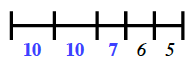### Home > MC1 > Chapter 5 > Lesson 5.2.2 > Problem5-57

5-57.

Find the length of each of the ropes shown in problem 5-56, given the following information.

1. In part (a), if $x = 20$ units, how long is the rope?

It may be helpful to look back at problem 4-123, since this problem is very similar to that one.

If the value of $x$ in the expression you wrote in problem 5-56 is $20$, can you find the total value? A diagram might be helpful here.

2. In part (b), if $j = 10$ units and $k = 7$ units, how long is the rope?

Use the re-labeled diagram below from problem 5-56 part (b) to help you solve this problem.